Question

In an experiment. it is possible to calculate the moles of oxygen from either the volume formed at STP or from the weight of oxygen generated. Which gives the more accurate value? Why?

Explain thee effect that failure to subtract the water vapor pressure from the total pressure would have on your determination of molar volume? (Would your molar volume be too high, the same, or too low and why?)

It is possible to calculate the moles of oxygen from the volume formed at STP gives the more accurate value than from the weight of oxygen generated.Because oxygen naturally occurs as gas at STP. So it is difficult to weigh the gas accurately as it needs bigger falsks. But we can measure the volume of gas accurately. So it is better to use the volume of gas to calculate the moles of gas.

Molar volume = moles/ volume of the gas

Volume of gas is high than original volume of gas because there is some amount of water vapor pressure. So we get low molar volume than original molar volume for gas.

Answer is your molar volume would be too low.

#### Earn Coins

Coins can be redeemed for fabulous gifts.

Similar Homework Help Questions
• ### Laboratory Report - The Molar Volume of a Gas Experiment 9: The Molar Volume of a...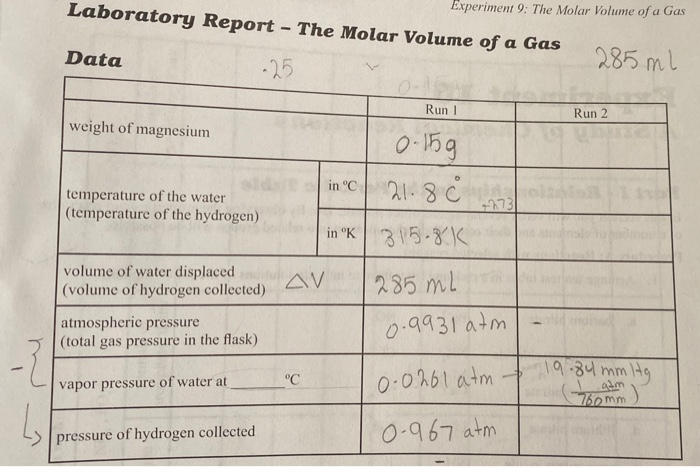Laboratory Report - The Molar Volume of a Gas Experiment 9: The Molar Volume of a Gas Data 285 ml Run 1 Run 2 weight of magnesium 0.15g incl 21. 8 Ĉ temperature of the water (temperature of the hydrogen) in °K 315-8 K volume of water displaced (volume of hydrogen collected) V 285 ML atmospheric pressure (total gas pressure in the flask) 0.9931 atm vapor pressure of water at 0.0261 atm 19.84 mm te pressure of hydrogen collected 0.967...

• ### What was the volume of N, corrected to STP Online 5. Calculate the moles of N,...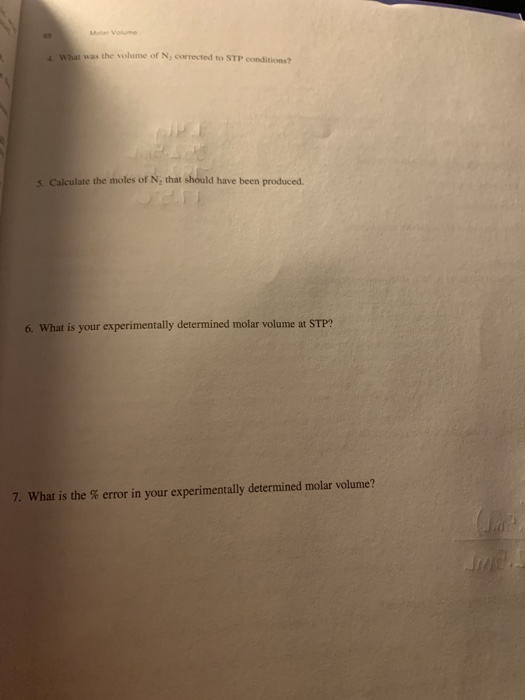What was the volume of N, corrected to STP Online 5. Calculate the moles of N, that should have been produced. 6. What is your experimentally determined molar volume at STP? 7. What is the % error in your experimentally determined molar volume? Molar Volume Name Section Instructor's Approval Volume of NaNO, solution used (show calculations in question 1) Mass of HSO,NH Volume of water to refill collection flask 1.419 352.5mL 782.5mm ty 17.50 Barometric pressure Water temperature Vapor pressure...

• ### TI Mass of empty tube after initial heating +8rotto 1.536 Mass of tube plus sample after...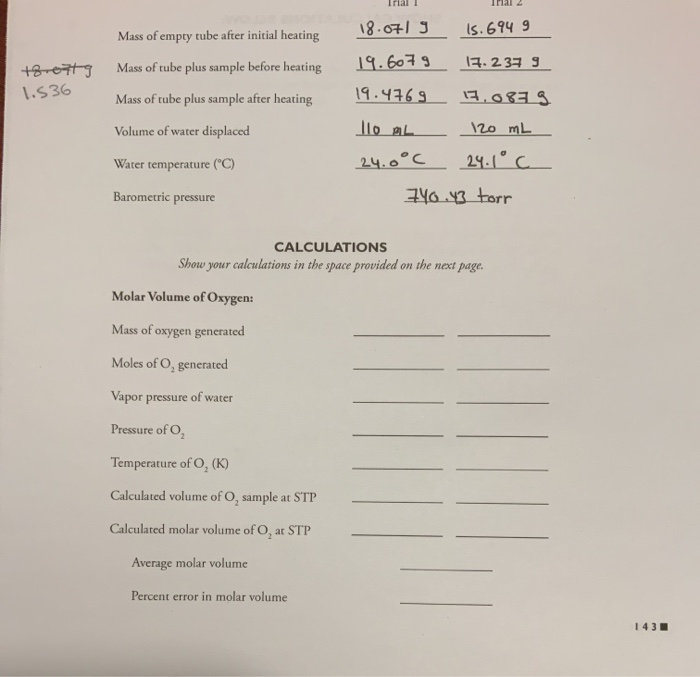TI Mass of empty tube after initial heating +8rotto 1.536 Mass of tube plus sample after heating 18.0719 15.694 9 19.6079 17.237 9 19.4769_13.0829 110 mL 120 mL 24.0°C 24.1°C 740.43 torr Volume of water displaced Water temperature (°C) Barometric pressure CALCULATIONS Show your calculations in the space provided on the next page. Molar Volume of Oxygen: Mass of oxygen generated Moles of O, generated Vapor pressure of water Pressure of Temperature of O, (K) Calculated volume of O, sample...

• ### Please help me with this lab I am so lost ! Inal T IS 3los Mass...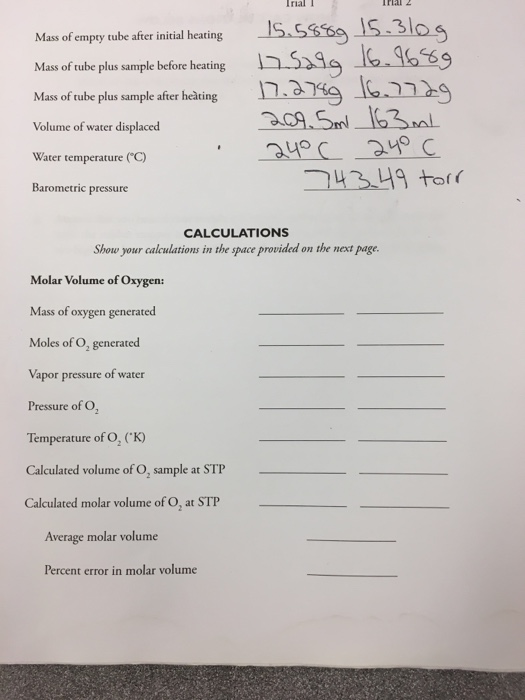Please help me with this lab I am so lost ! Inal T IS 3los Mass of empty tube after initial heating Mass of tube plus sample before heating Mass of tube plus sample after heating Volume of water displaced Water temperature ("C) n.a749 aup Barometric pressure CALCULATIONS Show your calculations in the space provided on the next page. Molar Volume of Oxygen: Mass of oxygen generated Moles of O, generated Vapor pressure of water Pressure of O2 Temperature of...

• ### The experiment this is in reference to is the determination of molar mass of a metal....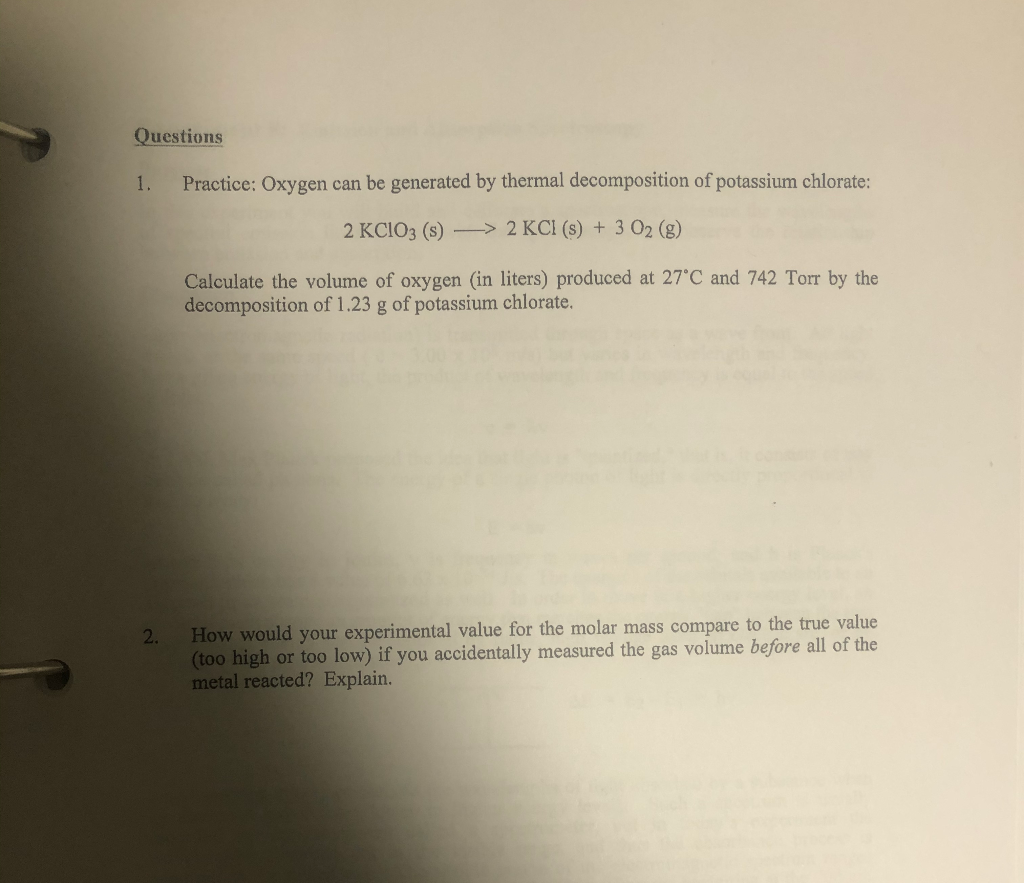The experiment this is in reference to is the determination of molar mass of a metal. a piece of magnesium ribbon was dangled on a copper wire into a tube with tap water and hydrochloric acid then placed into a beaker of water that was at room temperature. Questions 1. Practice: Oxygen can be generated by thermal decomposition of potassium chlorate: 2 KClO3 (s) —> 2 KCl (s) + 3 O2 (g) Calculate the volume of oxygen (in liters) produced...

• ### Analysis of a KClO3 mixture and the molar volume of Oxygen Gas

a 1.2475 g sample of a KCIO3 - KCI mixture is strongly heated. All of the KCI3 in the mixture undergoes decomposition. The potassium chloride from the originalsample plus the KCI generated by the reaction above weighed 1.0596 g. The gas generated from the reaction was collected over water and a volume of 192 mL at 24Cwas obtained. the barometric pressure was 645 mm Hg.a) dtermine the mass of O2 evolved by using gravimetric ( weight based ) measurments. Do...

• ### Two methods were descibed for determining the volume of the flask used for the molar mass...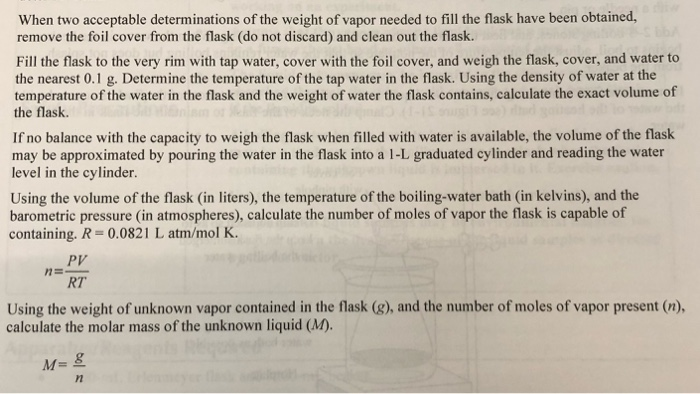Two methods were descibed for determining the volume of the flask used for the molar mass determination. Which method will give a more precise determination of the volume? Why? When two acceptable determinations of the weight of vapor needed to fill the flask have been obtained, remove the foil cover from the flask (do not discard) and clean out the flask. Fill the flask to the very rim with tap water, cover with the foil cover, and weigh the flask,...

• ### how do we find co2? Calculate 3. Complete the following data table, which is similar to...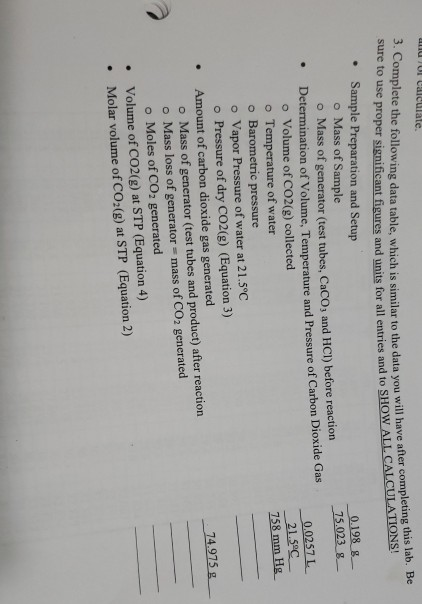how do we find co2? Calculate 3. Complete the following data table, which is similar to the data you will have a w use proper significant figures and units for all entries and to SHOW ALL CALL ar to the data you will have after completing this lab. Be nits for all entries and to SHOW ALL CALCULATIONS! • Sample Preparation and Setup o Mass of Sample 0.198_8_- o Mass of generator (test tubes, Caco, and HCI) before reaction 75.0238_...

• ### waa r the accepted value at SIF, and the perce REVIEW QUESTIONS MPC, rather than 45 ml of Me would have made any d...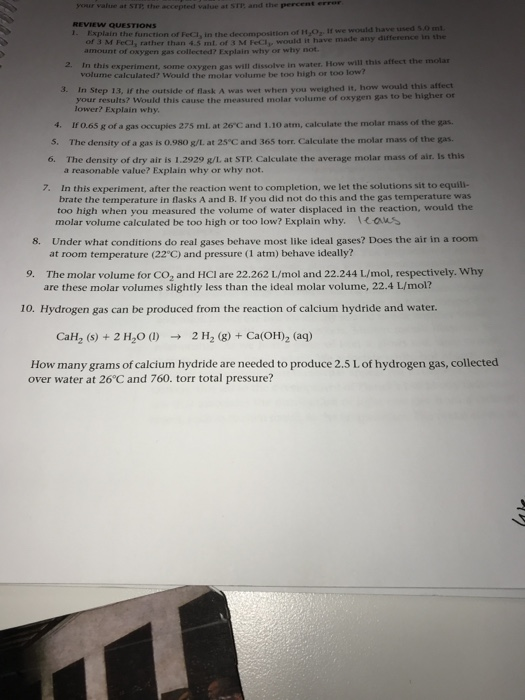waa r the accepted value at SIF, and the perce REVIEW QUESTIONS MPC, rather than 45 ml of Me would have made any difference in the amount of oxygen was collected? Explain why or why not In this experiment, some oxy en gas will dissolve in water How will this affect the molar volume calculated? Would the molar volume be too high or too low In Step 1, if the outside of flask was wet when you weighed it, how...

• ### Need help with the rest and how it was gotten Parts E and F I dont...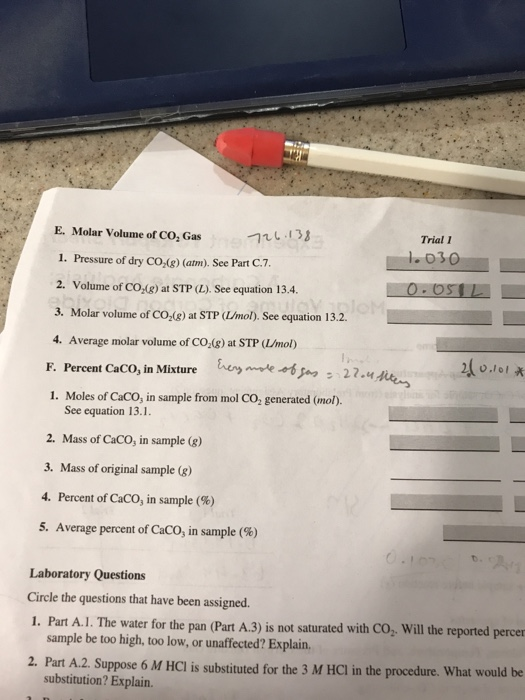Need help with the rest and how it was gotten Parts E and F I dont understand how to solve for them. the front page has all the data collected and what i was able to solve for E. Molar Volume of CO; Gas Trial I {nL 1.030 1. Pressure of dry CO,(g) (atm). See Part C.7 2. Volume of CO-(g) at STP (L). See equation 13.4. ulayanloM ebixo 3. Molar volume of CO,(g) at STP (Lmol). See equation 13.2....